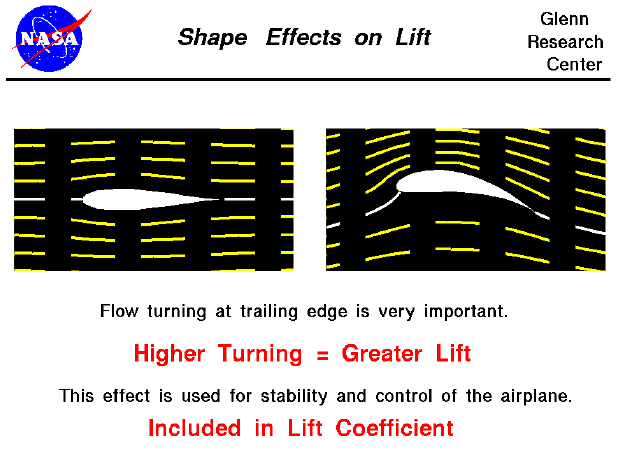The amount of lift generated depends on how much the flow is turned, which depends on the shape of the object. The lift is, in general, a very complex function of the shape. Aerodynamicists mathematically model the effect by a lift coefficient which is normally determined through wind tunnel testing. For certain simplified examples, however, we can develop mathematical equations for this dependence. Even the simplest example of shape dependence (two dimensional Kutta-Joukowski airfoil) is probably beyond the mathematical capabilities of high school students. However, the FoilSim program can provide the results of this analysis in a form readily usable by students. A simple result of the analysis shows that the greater the flow turning, the greater the lift.

This slide shows the flow fields for two different airfoils. The airfoil on the left is a symmetric airfoil; the shapes above and below the white centerline are the same. The airfoil on the right is curved near the trailing edge. The yellow lines on each figure show the streamlines of flow from left to right. The left figure shows no net turning of the flow and produces no lift; the right figure shows a large amount of turning and generates a large amount of lift. The front portions of both airfoils are nearly identical. The aft portion of the right airfoil creates the higher turning.

This explains why the aft portion of wings have hinged sections to control and maneuver an aircraft. Deflecting the aft section down will produce a geometry similar to the figure on the right producing more lift. Similarly, if the aft section is deflected up, it will create less lift (or even negative lift). The ability to vary the amount of lift over a portion of the wing gives the pilot the ability to maneuver an aircraft. The following slides show the deflection of the control surfaces and the resulting motion of the aircraft:

Let's investigate the dependence of lift on airfoil shape by using a Java simulator.

You can vary the shape of the foil by using the slider below the view window or by backspacing over the input box, typing in your new value and hitting the Enter key on the keyboard. On the right is a graph of the lift versus camber. The red dot shows your conditions. Below the graph is the numerical value of the lift. You can display either the lift value (in English or Metric units) or the lift coefficient by using the choice buttons surrounding the output box. Click on the choice button and select from the drop-menu.

As an experiment, set the camber to 0.0 per cent chord and note the amount of lift. Now increase the camber to 5 per cent. Did the lift increase or decrease? Set the camber to -5 per cent. What is the value of lift? Which way would this airfoil move?

You can further investigate the effect of airfoil shape and the other factors affecting lift by using the FoilSim II Java Applet. You can also download your own copy of FoilSim to play with for free.Guided Tours